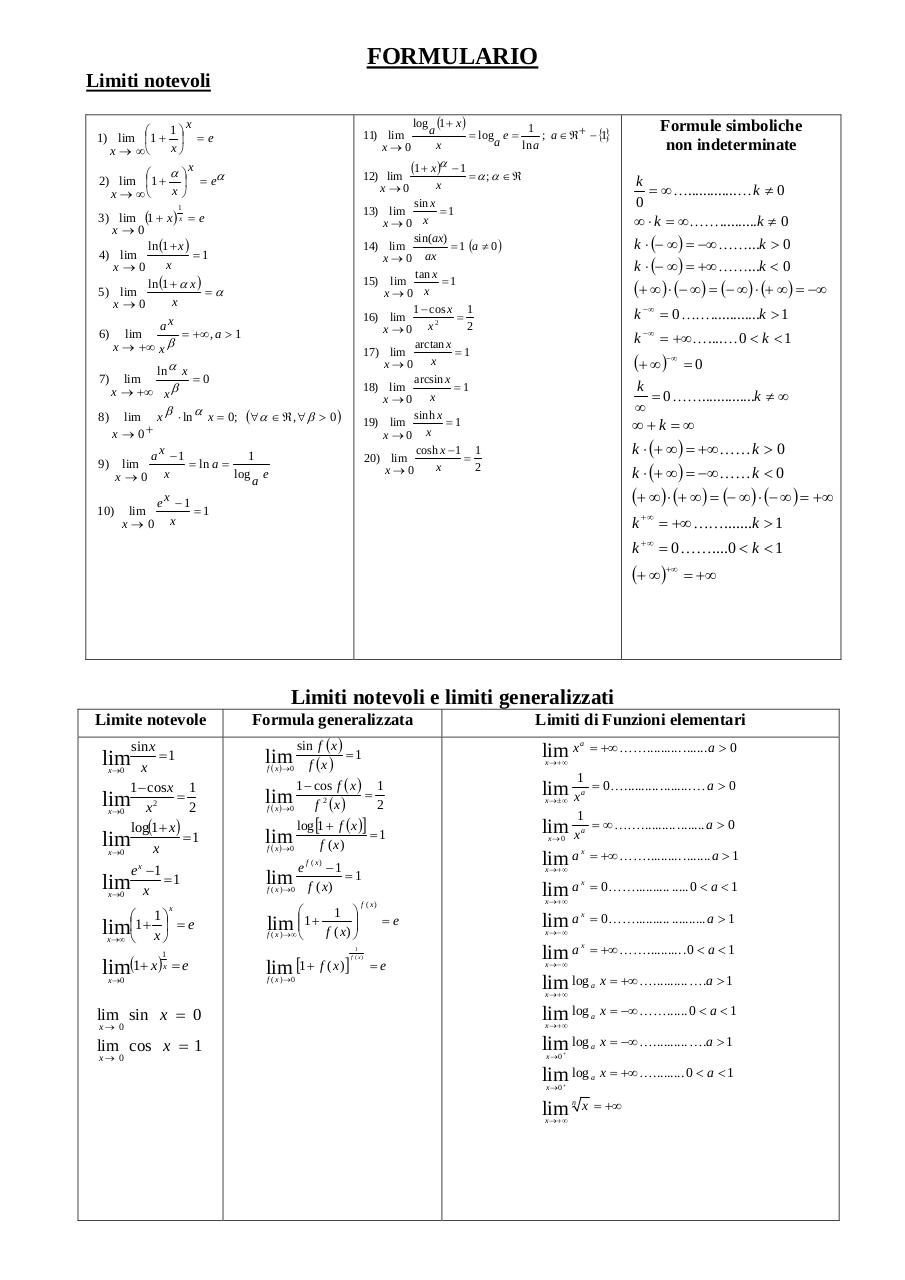# limiti notevoli .pdf

### File information

Original filename: limiti notevoli.pdf
Title: Microsoft Word - Formulario.doc
Author: GiÃ¹

This PDF 1.4 document has been generated by PScript5.dll Version 5.2.2 / Acrobat Distiller 7.0 (Windows), and has been sent on pdf-archive.com on 29/11/2014 at 16:42, from IP address 151.29.x.x. The current document download page has been viewed 10243 times.
File size: 30 KB (1 page).
Privacy: public file

limiti notevoli.pdf (PDF, 30 KB)

### Document preview

FORMULARIO
Limiti notevoli
1⎞

1) lim ⎜1 + ⎟
x⎠
x → ∞⎝

x

⎛ α⎞
2) lim ⎜1 + ⎟
x⎠
x → ∞⎝

=e

11) lim
x→0

= eα

12) lim
x→0

x

1

7)

lim
x → +∞ x β

=0

β

lim x ⋅ ln α x = 0; (∀α ∈ ℜ , ∀β &gt; 0 )
x → 0+
1
ax −1
= ln a =
9) lim
log
x
e
x→0
a

8)

10 )

lim
x→0

(1 + x)α
x

−1

= α; α ∈ ℜ

Formule simboliche
non indeterminate
k
= ∞ K.............K k ≠ 0
0
∞ ⋅ k = ∞ KK..........k ≠ 0
k ⋅ (− ∞ ) = −∞ KK...k &gt; 0

sin x
=1
13) lim
x→0 x
sin(ax)
= 1 (a ≠ 0)
14) lim
x → 0 ax
tan x
=1
15) lim
x→0 x
1 − cos x 1
=
16) lim
2
2
x→0 x
arctan x
=1
17) lim
x→0 x
arcsin x
=1
18) lim
x→0 x
sinh x
=1
19) lim
x→0 x
cosh x − 1 1
=
20) lim
2
x
x→0

3) lim (1 + x ) x = e
x→0
ln (1 + x )
=1
4) lim
x
x→0
ln (1 + α x )

5) lim
x
x→0
ax
= +∞ , a &gt; 1
6) lim
x → +∞ x β
ln α x

log (1 + x )
1
a
= log e =
; a ∈ ℜ + − {1}
a
x
ln a

k ⋅ (− ∞ ) = +∞ KK...k &lt; 0
(+ ∞ ) ⋅ (− ∞ ) = (− ∞ ) ⋅ (+ ∞ ) = −∞
k −∞ = 0 KK.............k &gt; 1
k −∞ = +∞ K....K 0 &lt; k &lt; 1

(+ ∞ )−∞ = 0

k
= 0 KK..............k ≠ ∞

∞+k =∞
k ⋅ (+ ∞ ) = +∞ KK k &gt; 0
k ⋅ (+ ∞ ) = −∞ KK k &lt; 0

(+ ∞ ) ⋅ (+ ∞ ) = (− ∞ ) ⋅ (− ∞ ) = +∞

ex −1
=1
x

k +∞ = +∞ KK.......k &gt; 1
k +∞ = 0 KK....0 &lt; k &lt; 1

(+ ∞ )+∞ = +∞

Limiti notevoli e limiti generalizzati
Limite notevole
sin x
=1
x
x→0
1 − cos x 1
=
lim
2
x2
x→0
log(1 + x)
=1
lim
x
x→0

lim

e x −1
=1
lim
x
x→0

1⎞

lim⎜⎝1+ x ⎟⎠

x

=e

x→∞

lim(1+ x)

1
x

=e

x→0

Formula generalizzata
sin f ( x )
=1
f (x )
x →0

lim x

lim
( )
f

log [1 + f ( x )]
=1
lim
f ( x)
f ( x )→ 0
e f ( x) − 1
=1
lim
f ( x)
f ( x )→0

f ( x )→ ∞

1 ⎞

f ( x ) ⎟⎠

lim [1 + f ( x )]
f ( x )→ 0

1

lim x
x → ±∞

a

1

lim x
x→0

a

x→ 0

lim cos x = 1
x→ 0

= ∞ KK .......... ........ a &gt; 0
= +∞ KK .......... ........ a &gt; 1

lim a

x

= 0 KK .......... ..... 0 &lt; a &lt; 1

lim a

x

= 0 KK .......... .......... a &gt; 1

x → −∞

lim a = +∞ KK.......... .0 &lt; a &lt; 1
lim log x = +∞ K.......... K.a &gt; 1
lim log x = −∞ KK...... 0 &lt; a &lt; 1
lim log x = −∞ K.......... K.a &gt; 1
x

=e

x → −∞

a

x → +∞

lim sin x = 0

= 0 K .......... ......... K a &gt; 0

x

x → +∞

=e

= +∞ KK .......... ....... a &gt; 0

lim a
x → +∞

f ( x)

1
f (x)

a

x → +∞

1 − cos f ( x ) 1
=
lim
2
f 2 (x )
f ( x )→ 0

lim ⎜⎜⎝1 +

Limiti di Funzioni elementari

a

x → +∞

a

x→0+

lim log
x→0+

lim
x → +∞

n

a

x = +∞ K ......... 0 &lt; a &lt; 1

x = +∞#### HTML Code

Copy the following HTML code to share your document on a Website or Blog

#### QR Code### Related keywords